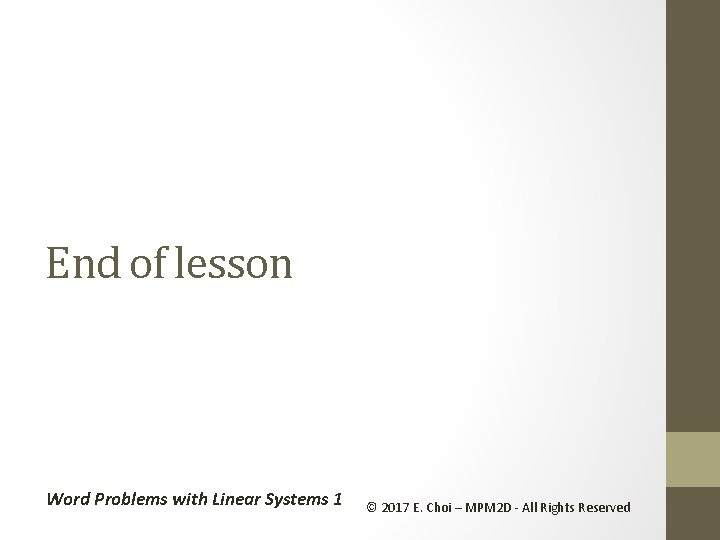• Slides: 8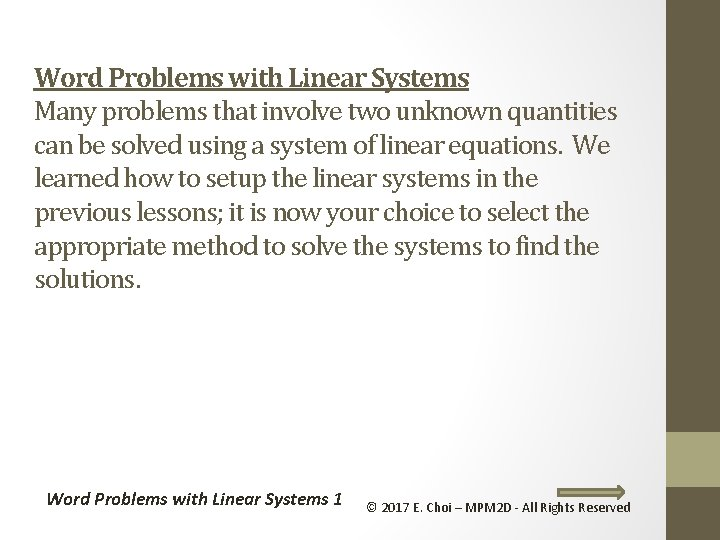Word Problems with Linear Systems Many problems that involve two unknown quantities can be solved using a system of linear equations. We learned how to setup the linear systems in the previous lessons; it is now your choice to select the appropriate method to solve the systems to find the solutions. Word Problems with Linear Systems 1 © 2017 E. Choi – MPM 2 D - All Rights Reserved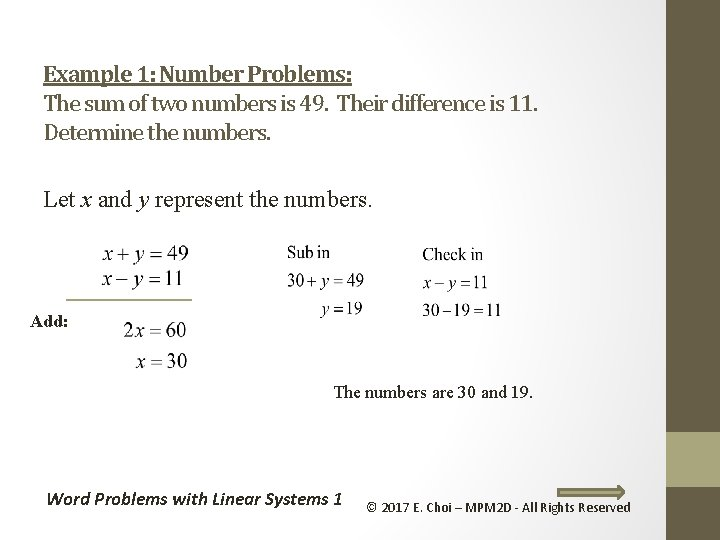Example 1: Number Problems: The sum of two numbers is 49. Their difference is 11. Determine the numbers. Let x and y represent the numbers. Add: The numbers are 30 and 19. Word Problems with Linear Systems 1 © 2017 E. Choi – MPM 2 D - All Rights Reserved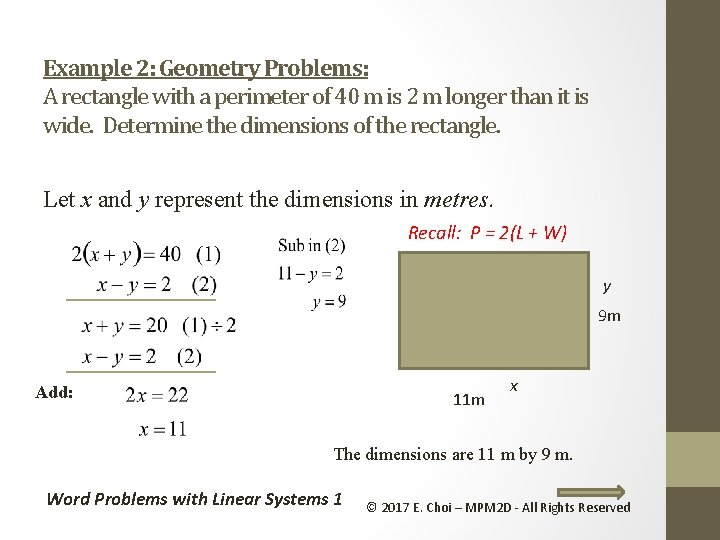Example 2: Geometry Problems: A rectangle with a perimeter of 40 m is 2 m longer than it is wide. Determine the dimensions of the rectangle. Let x and y represent the dimensions in metres. Recall: P = 2(L + W) y 9 m Add: 11 m x The dimensions are 11 m by 9 m. Word Problems with Linear Systems 1 © 2017 E. Choi – MPM 2 D - All Rights ReservedExample 3: Money Problems: Bob has a total of \$425 all in \$20 bills and \$5 bills. He has a total of 31 bills. How many \$20 bills does he have? Let x represent the number of \$20 bills Let y represent the number of \$5 bills Subtract: Bob has 18 twenty dollar bills … and 13 five dollar bills Word Problems with Linear Systems 1 © 2017 E. Choi – MPM 2 D - All Rights ReservedExample 4: Age problems. Sue is six years older than Joe. Four years ago she was twice as old as Joe was. How old is Joe now? Age Sue Joe Let x represent Sue’s current age Let y represent Joe’s current age Now x y 4 years ago From (1) Joe is 10 years old now and Sue is 16 years old now Word Problems with Linear Systems 1 © 2017 E. Choi – MPM 2 D - All Rights Reserved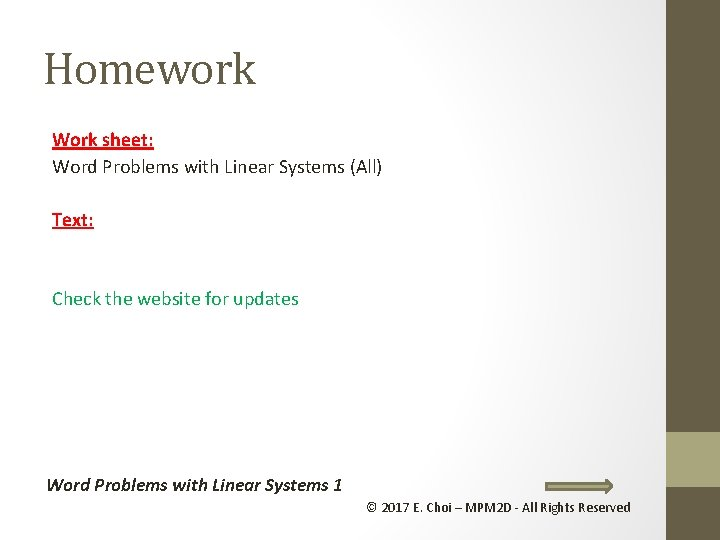Homework Work sheet: Word Problems with Linear Systems (All) Text: Check the website for updates Word Problems with Linear Systems 1 © 2017 E. Choi – MPM 2 D - All Rights Reserved